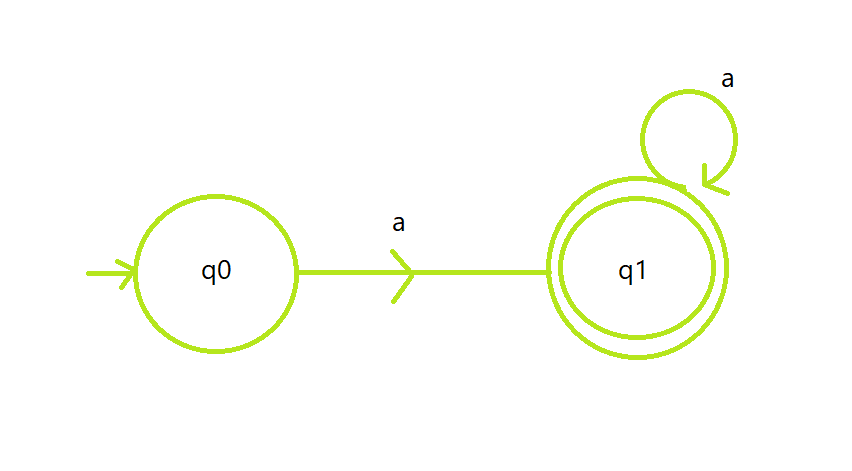Open in App
Not now

# Program to construct a DFA which accepts the language L = {aN | N ≥ 1}

• Last Updated : 28 Jun, 2021

Prerequisite: Finite Automata

Given a string S of size N, the task is to design a Deterministic Finite Automata (DFA) for accepting the language L = {aN | N ≥ 1}. The regular language L is {a, aa, aaa, aaaaaaa…, }. If the given string follows the given language L, then print “Accepted”. Otherwise, print “Not Accepted”.

Examples:

Input: S = “aaabbb”
Output: Not Accepted
Explanation: String must only contain a.

Input: S = “aa”
Output: Accepted

Approach: The idea by which the automata lead to acceptance of string is stated below in steps:

• The automata will accept all the strings containing only the character ‘a’. If the user tried to input any character other than ‘a’, the machine will reject it.
• Let the state q0 is the initial state represent the set of all strings of length 0, state q1 is the final state represent the set of all strings from 1 to N.
• State q1 contains a self-loop of a which indicates that it can be repeated as required.
• The logic for code is very basic as it has only a for loop which counts the number of a’s in a given string, if the count of a is the same as N then it will be accepted. Otherwise, the string will be rejected.

DFA State Transition Diagram:Below is the implementation of the above approach:

## C++

 `// C++ program for the above approach` `#include ``using` `namespace` `std;` `// Function to check whether the string``// S satisfy the given DFA or not``void` `isAcceptedDFA(string s, ``int` `N)``{``    ``// Stores the count of characters``    ``int` `count = 0;` `    ``// Iterate over the range [0, N]``    ``for` `(``int` `i = 0; i < N; i++) {` `        ``// Count and check every``        ``// element for 'a'``        ``if` `(s[i] == ``'a'``)``            ``count++;``    ``}` `    ``// If string matches with DFA``    ``if` `(count == N && count != 0) {``        ``cout << ``"Accepted"``;``    ``}` `    ``// If not matches``    ``else` `{``        ``cout << ``"Not Accepted"``;``    ``}``}` `// Driver Code``int` `main()``{``    ``string S = ``"aaaaa"``;` `    ``// Function Call``    ``isAcceptedDFA(S, S.size());` `    ``return` `0;``}`

## Java

 `// Java program for the above approach``class` `GFG``{` `// Function to check whether the String``// S satisfy the given DFA or not``static` `void` `isAcceptedDFA(String s, ``int` `N)``{``    ``// Stores the count of characters``    ``int` `count = ``0``;` `    ``// Iterate over the range [0, N]``    ``for` `(``int` `i = ``0``; i < N; i++)``    ``{` `        ``// Count and check every``        ``// element for 'a'``        ``if` `(s.charAt(i) == ``'a'``)``            ``count++;``    ``}` `    ``// If String matches with DFA``    ``if` `(count == N && count != ``0``)``    ``{``        ``System.out.print(``"Accepted"``);``    ``}` `    ``// If not matches``    ``else``    ``{``        ``System.out.print(``"Not Accepted"``);``    ``}``}` `// Driver Code``public` `static` `void` `main(String[] args)``{``    ``String S = ``"aaaaa"``;` `    ``// Function Call``    ``isAcceptedDFA(S, S.length());``}``}` `// This code is contributed by 29AjayKumar`

## Python3

 `# Python3 program for the above approach` `# Function to check whether the string``# S satisfy the given DFA or not``def` `isAcceptedDFA(s, N):``  ` `    ``# Stores the count of characters``    ``count ``=` `0` `    ``# Iterate over the range [0, N]``    ``for` `i ``in` `range``(N):` `        ``# Count and check every``        ``# element for 'a'``        ``if` `(s[i] ``=``=` `'a'``):``            ``count ``+``=` `1` `    ``# If string matches with DFA``    ``if` `(count ``=``=` `N ``and` `count !``=` `0``):``        ``print` `(``"Accepted"``)` `    ``# If not matches``    ``else` `:``        ``print` `(``"Not Accepted"``)` `# Driver Code``if` `__name__ ``=``=` `'__main__'``:``    ``S ``=` `"aaaaa"` `    ``# Function Call``    ``isAcceptedDFA(S, ``len``(S))` `# This code is contributed by mohit kumar 29`

## C#

 `// C# program for the above approach``using` `System;``class` `GFG``{` `// Function to check whether the String``// S satisfy the given DFA or not``static` `void` `isAcceptedDFA(String s, ``int` `N)``{``  ` `    ``// Stores the count of characters``    ``int` `count = 0;` `    ``// Iterate over the range [0, N]``    ``for` `(``int` `i = 0; i < N; i++)``    ``{` `        ``// Count and check every``        ``// element for 'a'``        ``if` `(s[i] == ``'a'``)``            ``count++;``    ``}` `    ``// If String matches with DFA``    ``if` `(count == N && count != 0)``    ``{``        ``Console.Write(``"Accepted"``);``    ``}` `    ``// If not matches``    ``else``    ``{``        ``Console.Write(``"Not Accepted"``);``    ``}``}` `// Driver Code``public` `static` `void` `Main(String[] args)``{``    ``String S = ``"aaaaa"``;` `    ``// Function Call``    ``isAcceptedDFA(S, S.Length);``}``}` `// This code is contributed by 29AjayKumar`

## Javascript

 ``

Output:

`Accepted`

Time Complexity: O(N)
Auxiliary Space: O(1)

My Personal Notes arrow_drop_up